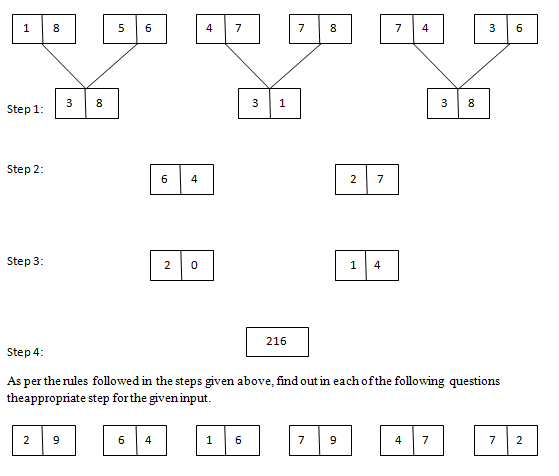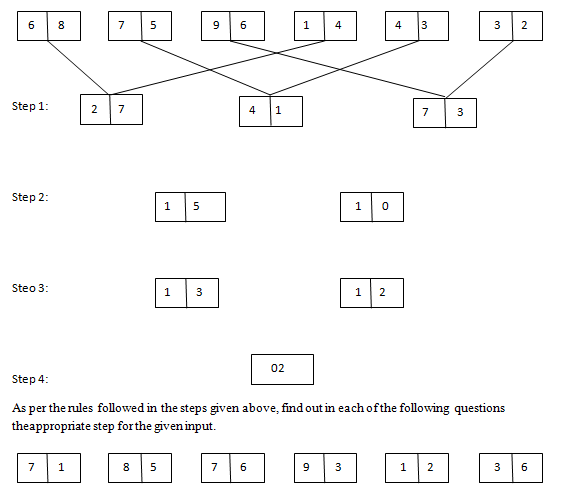# Reasoning – New Pattern Input Output Questions Day-4

Reasoning – New Pattern Input Output Questions Day-4:

Dear Readers, We all knew that IBPS has introduced some new pattern Reasoning Questions in the Examinations. Many of our followers were requesting us to provide Practice Questions based on the new pattern, so for that we have started providing input and output reasoning questions. Kindly follow us regularly and make use of it, if you have any suggestion kindly use the comment section below.

[WpProQuiz 755]

### Click “Start Quiz” to attend these Questions and view Solutions

Directions (1-5): Study the given information carefully and answer the given questions.

An input-output is given in different steps. Some mathematical operations are done in each step. No mathematical operation is repeated in next step.1) If the 1st block is divided by ‘5’ and the 2nd block is divided by ‘6’ in step 2, then what will be the sum of two blocks?

a)23

b)24

c)21

d)18

e)None of these

2) If the 2nd block in step 3 is divided by ‘3’, then what will be the square root of the value?

a)1

b)2

c)3

d)4

e)None of these

3) What will be the difference between the sum of two digits in block 1 and sum of two digits in block 2 in step 2?

a)4

b)2

c)1

d)3

e)5

4) What will be the difference between cube value of 2nd digit of 2nd block in step 2 and 1st digit of 2nd block in step 1?

a)210

b)206

c)226

d)222

e)None of these

5) Which of the following represents the product of cube root of final step and square root of 2nd block in 2nd step?

a)15

b)16

c)14

d)17

e)18

Directions (6-10): Study the given information carefully and answer the given questions.

An input-output is given in different steps. Some mathematical operations are done in each step. No mathematical operation is repeated in next step.6) What is the difference between 2nd block of 2nd step and 1st block of 3rd step?

a)8

b)10

c)20

d)2

e)None of these

7) If in step 1, odd digits are added with ‘1’ and even digits are subtracted with ‘2’, then what will be the sum of 2nd digit of 1st block and 1st digit of 2nd block?

a)8

b)10

c)6

d)4

e)None of these

8) Which of the following represents the 2nd digit of 2nd block in step 2 and 1st digit of the final step respectively?

a)2, 5

b)3, 2

c)7, 4

d)5, 2

e)2, 7

9) If the 1st block in step 1 is divided by the final step and added to the 1st block in step 3, then the answer will represent the value in?

a)2nd block in step 2

b)2nd block in step 3

c)2nd block in step 1

d)3rd block in step 1

e)final step

10) What is the product of 2nd block in step 2 and 1st block in step 3?

a)525

b)625

c)425

d)725

e)325

• #### Reasoning Input Output New Pattern Questions – Day-3